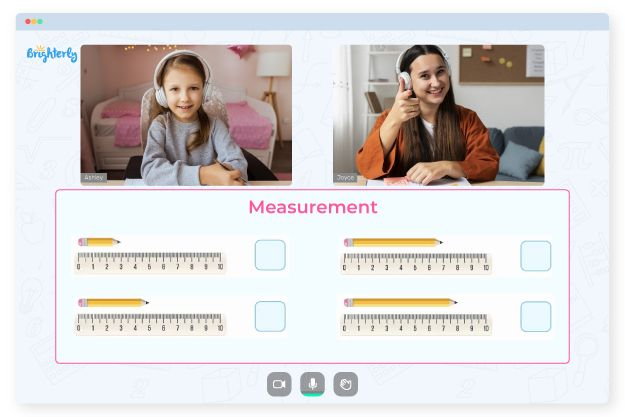# Measuring To The Nearest 1/2 Inch Worksheets

Measuring to the nearest half inch worksheets contain activities that teach kids to round a length measurement to the nearest half inch increment. A measuring to the half inch worksheet makes the process of measurements more understandable and faster, reducing scale errors.

## How It Works

To find the nearest half inch measurement, kids need to measure the length using a ruler or measuring tape and round the measurement to the nearest half inch. For example, if the length measured is 2.3 inches, the closest half inch size would be 2.5 inches. If the length measured 2.6 inches, the nearest half inch measurement would be 2.5 inches.

Math for Kids

Is Your Child Struggling With Math?
1:1 Online Math Tutoring## Benefits

There are a few potential benefits of using a measure to the nearest 1/2 inch worksheet. Taking measurements becomes faster since kids only need to look at a ruler or measure tape to the nearest half inch rather than trying to measure to the exact fraction of an inch. Measure to the nearest half inch worksheets also reduce measurement errors since measuring to tiny fractions of an inch can be difficult.### Measuring To The Nearest 1/2 Inch Worksheets PDF

Measuring To The Nearest 1/2 Inch Worksheet### Measuring To The Nearest 1/2 Inch Worksheets PDF

Measure To The Nearest 1/2 Inch Worksheet### Measuring To The Nearest 1/2 Inch Worksheets PDF

Measuring To The Nearest Half Inch Worksheets### Measuring To The Nearest 1/2 Inch Worksheets PDF

Measuring To The Nearest Half Inch Worksheet

Additionally, measuring to the nearest half inch worksheet allows kids to simplify calculations since half inch increments are more managea ble to work with than smaller increments.

### More Measurement Worksheets

Trouble with Measurement?• Does your child struggle with grasping measurement? An online tutor could help.

Is your child finding it challenging to understand measurement? Start lessons with an online tutor.